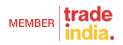# Heavy Duty Mixer Grinder

## 5 Ltr Tilting Mixer

Price: 18000 INR/Piece

Capacity = 5 Ltr Power = 1.5 HP Weight = 36 Kg

## 3 Ltr Mixer Machine Round Model

Price: 11000 INR/Piece

Capacity = 3 Ltr Power = 1.5 HP Weight = 27 Kg Speed = 2880 RPM

## 14 Ltr Mixer Machine Square Model

Price: 22000 INR/Piece

Capacity = 14 Ltr Power = 3 HP Weight = 45 Kg

## 14 Ltr Mixer Machine Round Model

Price: 22000 INR/Piece

Capacity = 14 Ltr Power = 3 HP Weight = 27 Kg

## 5 Ltr Mixer Machine Square Model

Price: 17000 INR/Piece

Capacity = 5 Ltr Power = 1.5 HP Weight = 35 Kg

## 10 Ltr Mixer Machine Square Model

Price: 21000 INR/Piece

Capacity = 10 Ltr Power = 2 HP Weight = 40 Kg

## 10 Ltr Tilting Mixer

Price: 22000 INR/Piece

Capacity = 10 Ltr Power = 2 HP Weight = 41 Kg

## 14 Ltr Tilting Mixer

Price: 24000 INR/Piece

Capacity = 14 Ltr Power = 3 HP Weight = 51

## 1HP V Belt Mixer Machine

Price: 25000 INR/Piece

Power = 1 HP Speed = 1440 RPM Weight = 45 Kg

## 1.5 HP V Belt Mixer Machine

Price: 32000 INR/Piece

Power = 1.5 HP Speed = 1440 RPM Weight = 50 Kg

## 2HP Three Phase V Belt Mixer Machine

Price: 40000 INR/Piece

Power = 2 HP Speed = 1440 RPM Weight = 60 Kg

## 2 Ltr Mixer Machine Round Model

Price: 10000 INR/Piece

Capacity = 2 Ltr Power = 0.5 HP Weight = 11 Kg Speed = 2880 RPM

## 5 LTR 1.5 HP Tilting Mixer

Price: 18000 INR/Piece

Moc = SS Weight = 36 Kg Speed = 2880 RPM

## 10 Ltr Mixer Machine Round Model

Price: 17000 INR/Piece

Capacity = 10 Ltr Power = 2 HP Weight = 37 Kg

XREIGNITECH EXIM PRIVATE LIMITED All Rights Reserved.(Terms of Use)Developed and Managed by Infocom Network Ltd.